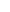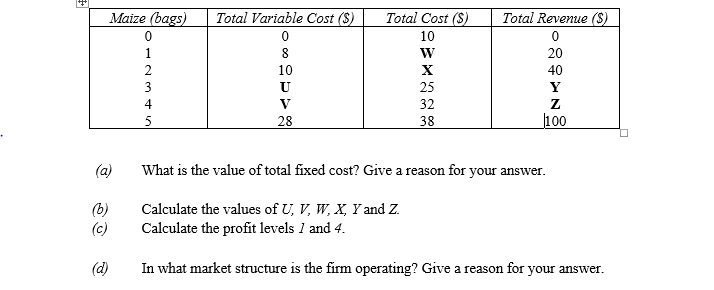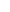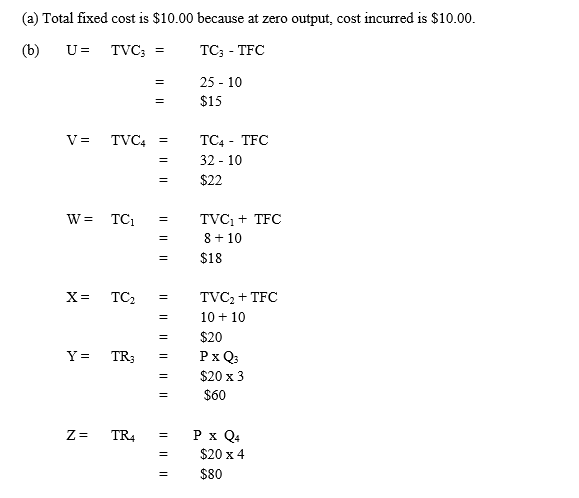Question 1The table below shows the cost of production and output of maize. The price of maize is fixed \$20.00. Use the information to answer the questions that follow.Observation

This is one of the data response question and was not popular with candidates. The few who attempted the question scored above average marks. The question required candidates to determine the value of the fixed cost of the firm, calculate the values of some variables, calculate profits at some output levels and determine what market structure the firm was operating in the (a) – (d)  parts of the question respectively. Most candidates who attempted this question were able to calculate the values of the variables and the profit levels but only few were able to determine the market structure the firm was operating in the (d) part of the question.
The candidates were expected to provide the following answers to score higher marks.(c)        Profit is TR – TC
At output level 1, profit    =    \$20 - \$18
=    \$2.00
At output level 4, profit    =    \$80.00 - \$32.00
=    \$48.00

(d)       Perfect competition/market because price is fixed at \$20.00